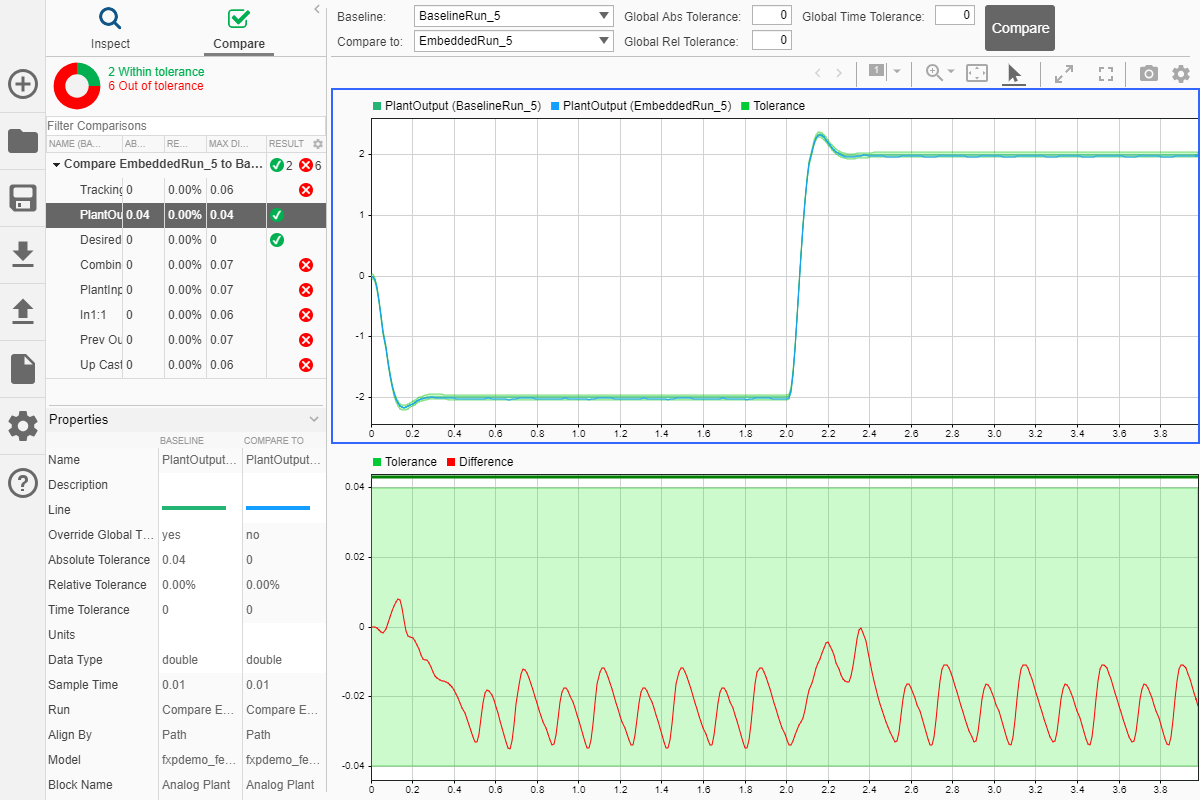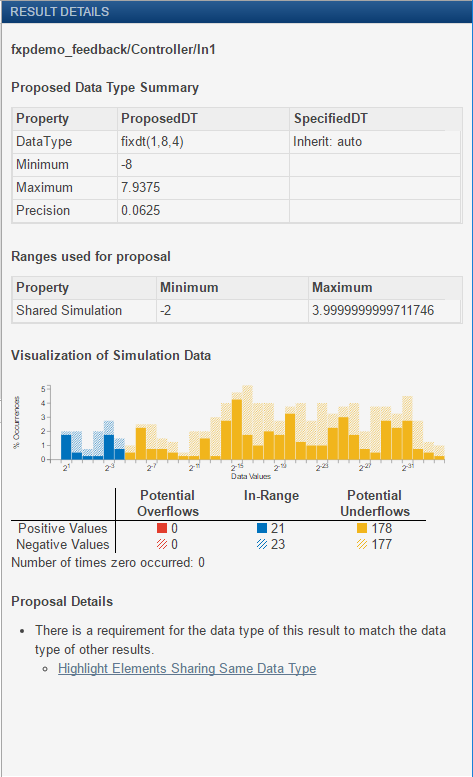## View Simulation Results

The Fixed-Point Tool uses the Simulation Data Inspector tool plotting capabilities that enable you to plot logged signals for graphical analysis. Using the Simulation Data Inspector to inspect and compare data after converting your floating-point model to fixed point facilitates tracking numerical error propagation.

Use the Simulation Data Inspector to:

• Plot multiple signals in one or more axes

• Compare a signal in different runs

• Compare all logged signal data from different runs

• Export signal logging results to a MAT-file

• Specify tolerances for signal comparison

• Create a report of the current view and data in the Simulation Data Inspector

### Compare Runs

To compare runs, in the Fixed-Point Tool, right-click on the embedded run and select Open SDI.

On the upper axes, the Simulation Data Inspector plots the signal for the selected runs and the tolerance, if specified. On the lower axes, the Simulation Data Inspector plots the difference between those runs.### Histogram Plot of Signal

To view the histogram plot of a signal, select the signal in the Results spreadsheet. The Result Details pane includes a histogram plot that helps you visualize the dynamic range of a signal. It provides information about the:

• Total number of samples (N).

• Maximum number of bits to prevent overflow.

• Number of times each bit has represented the data (as a percentage of the total number of samples).

• Number of times that exact zero occurred (without the effect of quantization). This number does not include the number of zeroes that occurred due to rounding.

You can use this information to estimate the word size required to represent the signal.## SupportGet trial now